• #include #define Height 10 ... printf("长方形的体积是:"); printf("%d\n",result); return 0; } int calculate(int Long,int Width) { int result=Long*Width*Height; return result; }
• #include<stdio.h> #include<math.h> #include<windows.h>... printf("________长方体体积计算（单位：厘米）_______\n\n"); float a; printf("请输入长：\n"); scanf(.
#include<stdio.h>
#include<math.h>
#include<windows.h>
int main()
{
int i;
for (i=0; i >= 0; i++)
{
int i;
for (i=0; i < 5; i++)
{
printf("________长方体体积计算（单位：厘米）_______\n\n");
float a;
printf("请输入长：\n");
scanf("%f", &a);
float b;
printf("请输入宽：\n");
scanf("%f", &b);
float c;
printf("请输入高：\n");
scanf("%f", &c);

printf("长:%f\n", a);
printf("宽:%f\n", b);
printf("高:%f\n", c);

float v;
v=a*b*c;
printf("************************\n");
printf("*长方体的体积为：%f立方厘米 *\n", v);
printf("************************\n\n");
getchar();
}
system("cls");
}
return 0;
}

在c-free5和dev c++上都能运行
展开全文• 计算长方体体积 有朋友会说长方体体积还不好算吗？长X宽X高。没错用计算器一下就可以出结果，编程反而麻烦些，但是我们说的是这种思维，如果复杂的重复运算的话写好程序就非常简单了. 简单运算下一个固定高度的...
计算长方体体积
有朋友会说长方体体积还不好算吗？长X宽X高。没错用计算器一下就可以出结果，编程反而麻烦些，但是我们说的是这种思维，如果复杂的重复运算的话写好程序就非常简单了.
简单运算下一个固定高度的长方体体积计算，先看下运行后面板.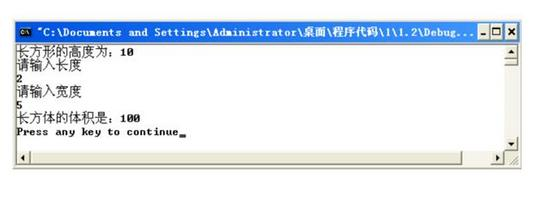长度和宽度是可以自由输入的哦
那么这个程序该怎么编写？
第一步先把头文件写上
C++/C程序的头文件以“.h”为后缀。
stdio.h
stdio.h就是指“standard input&output" 意思就是说标准输入输出头文件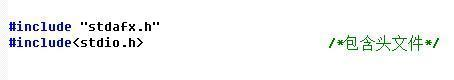大家一起动手写写试试
第二步定义常量
先给他定义一个固定的高度，我们给他定为10.（define，无参宏定义的一般形式为:#define 标识符 字符串
define 函数定义一个常量常量类似变量，不同之处在于在设定以后，常量的值无法更改。）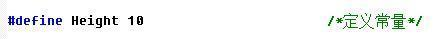第三步函数声明
函数声明是为了让下面调用函数的那个地方认识你被调用的函数。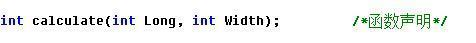第四步主函数
这个没什么好说的main函数，大家发现这里经常出现int。int是关键字： 声明整型变量或函数！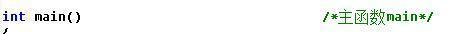记得后面加（）哦
第五步函数体
主函数中{}中的内容被称为函数体
1.定义整型变量
因为前面定义过高了所以这里只需定义长，宽和体积就可以了，取名字最好取个意思相关的。例如：长定义为m_long,长的英文，如果定义别的可能当时能分辨出来后期再看就会忘了定义的是什么了，所以一定要养成良好的习惯。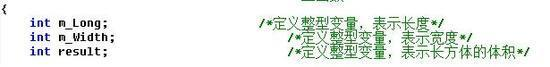2.输出函数
printf 表示输出函数，这里的输出就是运行后的显示结果。先看一下实例：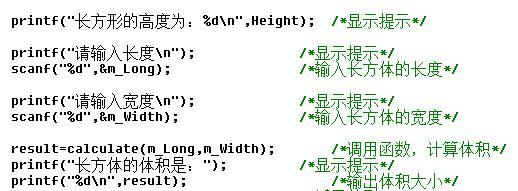printf("长方形的高度为：%d ",Height);
长方形的高度为，运行后直接显示
"%d"输入输出为整型。
" "换行。
Height放在这个位置就是调用上面定义的这个词.上面定义的是10，所以这里显示10.
这句话运行后显示为下图：scanf() 是C语言中的一个输入函数。与printf函数一样，都被声明在头文件stdio.h里，因此在使用scanf函数时要加上#include 。它是格式输入函数，即按用户指定的格式从键盘上把数据输入到指定的变量之中。
上图中这些输出函数意思都差不多就不一 一讲解了
3.返回值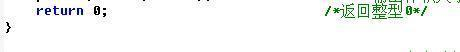返回整型0
这里返回结果为什么是0？因为上面那些只是定义了常量，变量和输出还没有定义怎么运算
第六步定义体积函数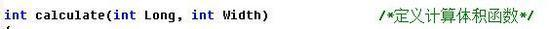怎么样看着眼熟？是不是和上面的函数声明长得很像？NO!NO!NO!本来就是一样的【捂脸】虽然一样的但是这里还是要再写一次，因为这个已经不再是上面那个函数体中的了。这是一个新的函数体所以要再定义一下.然后函数体还要写在{}中哦
第七步运算公式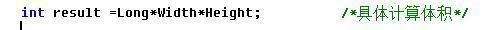这个就好理解了体积等=长*宽*高
第八步将计算结果返回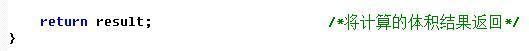将计算的 result 的值返回到上面main主函数输出的 result 中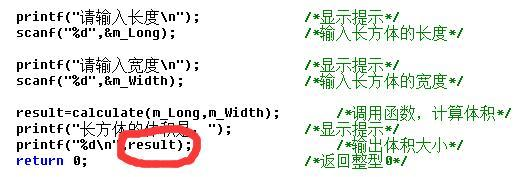整个程序写完就是这样的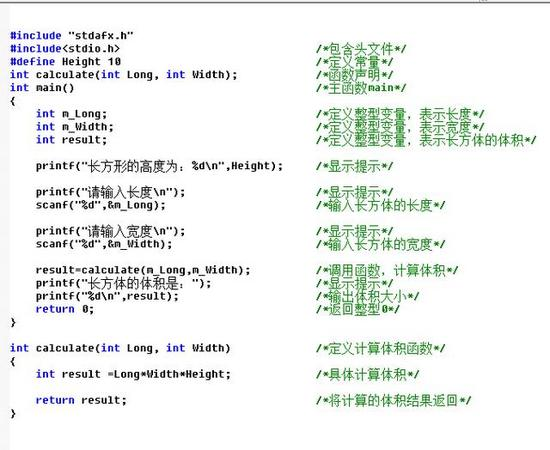这个不难的大家自己试试写写看！
——————————————
看到这里你是不是对数据结构又有了一点新的认知呢~
如果你喜欢这篇文章的话，动动小指，点个赞再走~
如果你想学编程，小编推荐一个C语言/C++编程学习基地【点击进入】！一个活跃、高逼格、高层次的编程学习殿堂；编程入门只是顺带，思维的提高才有价值！
涉及：编程入门、游戏编程、网络编程、Windows编程、Linux编程、Qt界面开发、黑客等等....
展开全文• （2）计算长方体体积；3）输出3个长方体的体积。二、建立一个对象数组，内放5个学生的数据（学号、成绩）。（1）用指针指向数组首元素，输出第1、3、5个学生的数据；2）设立一个函数max，用指向对象的指针作函数...
目录
一、需要求3个长方体的体积，请编一个基于对象的程序。数据成员包括length（长）、width（宽）、height（高）。要求同成员函数实现以下功能。
二、建立一个对象数组，内放5个学生的数据（学号、成绩）。
三、商店销售某一商品，商店每天公布统一的折扣（discount），同时允许销售人员在销售时灵活掌握售价（price）。在此基础上，对一次购10件以上的顾客，还可以享受9.8折优惠。现已知当天有3个销货员的销售情况为：

一、需要求3个长方体的体积，请编一个基于对象的程序。数据成员包括length（长）、width（宽）、height（高）。要求同成员函数实现以下功能。
（1）由键盘分别输入3个长方体的长、宽、高；
（2）计算长方体的体积；
（3）输出3个长方体的体积。
思路
该题目中长方体类只有一个计算体积的成员函数，于是使用内联函数的方式实现。分别获取三个长方体的长、宽、高，用于初始化长方体对象，然后调用函数输出长方体体积。
参考代码
#include <iostream>
using namespace std;

class Rectangle{
public:
Rectangle(float l,float w,float h){  //构造函数
length = l; width = w; height = h;
}
float volume(){ //求体积函数
return length*width*height;
}
private:
float length,width,height;
};

int main(){
float al,aw,ah,bl,bw,bh,cl,cw,ch,av,bv,cv;
//获取长方体a的长宽高
cout << "------- a -------" << endl;
cout << "Input length:";
cin >> al;
cout << "Input width:";
cin >> aw;
cout << "Input height:";
cin >> ah;
//获取长方体b的长宽高
cout << "------- b -------" << endl;
cout << "Input length:";
cin >> bl;
cout << "Input width:";
cin >> bw;
cout << "Input height:";
cin >> bh;
//获取长方体c的长宽高
cout << "------- c -------" << endl;
cout << "Input length:";
cin >> cl;
cout << "Input width:";
cin >> cw;
cout << "Input height:";
cin >> ch;
Rectangle a(al,aw,ah);
Rectangle b(bl,bw,bh);
Rectangle c(cl,cw,ch);
cout << "\n ---- volume ----" <<endl;
cout << "a: " << a.volume() << endl; //输出长方体a的体积
cout << "b: " << b.volume() << endl; //输出长方体b的体积
cout << "c: " << c.volume() << endl; //输出长方体c的体积
return 0;
}

结果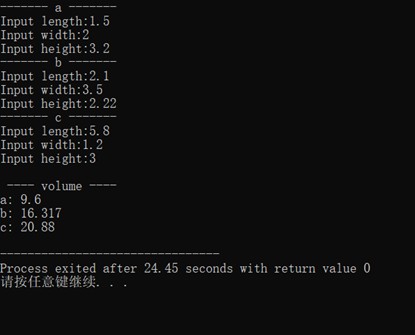二、建立一个对象数组，内放5个学生的数据（学号、成绩）。
（1）用指针指向数组首元素，输出第1、3、5个学生的数据；
（2）设立一个函数max，用指向对象的指针作函数参数。在max函数中找出5个学生中学生成绩最高者，并输出其学号。
思路
建立一个对象数组，用指针指向其首地址，移动指针输出对象；函数Max以对象指针为参数，比较成绩找到最高者并输出。使用了参数初始化表初始化；主要需要注意对象数组建立，实际上是同时实例化一组对象。
参考代码
#include <iostream>
using namespace std;

class Student{
public:
Student(string num,float s):number(num),score(s){}; //使用参数初始化表初始化数据成员
void display(); //输出学生信息函数
friend void max(Student *p);
private:
string number; //学号
float score; //成绩
};
void Student::display(){
cout << "学号：" << number << endl;
cout << "成绩：" << score << endl;
cout << "------------"<< endl;
}
void max(Student *p){ //指向对象的指针作参数
Student *temp = p;
for(int i=0;i<5;i++)
if(temp->score<(temp+i)->score)
temp+=i;
cout << "成绩最高者为："<< temp->number << endl; //输出成绩最高者学号
}

int main(){
Student Stu[]={Student("2018215112",96),Student("2018215110",98),Student("2019214120",87),Student("2019211124",95),Student("2019222111",66)};
Student *p = Stu;
for(p=Stu;p<Stu+5;p+=2)
p->display();
max(Stu);
}

结果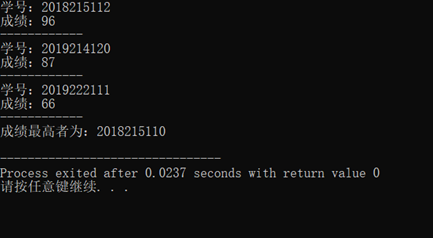三、商店销售某一商品，商店每天公布统一的折扣（discount），同时允许销售人员在销售时灵活掌握售价（price）。在此基础上，对一次购10件以上的顾客，还可以享受9.8折优惠。现已知当天有3个销货员的销售情况为：
销货员号（num）  销货件数（quantity）  销货单价（price）  101  5  23.5  102  12  24.56  103  100  21.5
请编程，计算出当日此商品的总销售额sum以及每件商品的平均售价。
要求用静态数据成员和静态成员函数（提示：将折扣discount、总销售额sum、商品销售总件数n声明为静态数据成员；再定义静态成员函数average（求平均售价）和display（输出结果））
思路
使用对象数组实例化三个对象，在类中定义了静态成员变量和静态成员函数让数据为所有对象共享。每个对象调用total函数计算总销售额和总件数；求平均售价。要注意静态成员函数必须调用静态成员变量。
参考代码
#include <iostream>
using namespace std;

class Commodity{
public:
Commodity(int n,int q,float p):num(n),quantity(q),price(p){} //参数初始化表初始化
void total(); //求总销售额和总销售件数函数
static float average(); //求平均售价的静态成员函数
static void display(); //输出总销售额和平均售价的静态成员函数
private:
int num;
int quantity;
float price;
static int n;
static float discount,sum;
};

int Commodity::n = 0;
float Commodity::discount = 0.95;
float Commodity::sum = 0; //静态成员必须赋初始值

void Commodity::total(){
float rate = 1.0;
if(quantity>10)
rate = 0.98;
sum = sum + price*quantity*rate*discount; //求总销售额
n = n+quantity; //求总销售件数
}

float Commodity::average(){
return (sum/n);  //求平均售价
}

void Commodity::display(){ //输出总销售额和平均售价
cout << "总销售额：" << sum << endl;
cout << "平均售价：" << average() << endl;
}

int main(){
Commodity Com = {Commodity(101,5,23.5),Commodity(102,12,24.56),Commodity(1.3,100,21.5)}; //使用对象数组存放三个销售员情况
for(int i=0;i<3;i++)
Com[i].total(); //对于每个对象调用total函数，统计三个销售员的情况
Commodity::display(); //输出最终结果
return 0;
}

结果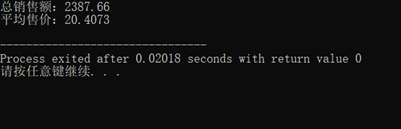四、定义一个复数类Complex，重载运算符“+”使之能用于复数之间的加法运算。参加运算的两个运算量都可以是类对象，也可以其中一个是整数，顺序任意。例如：c1+c2,i+c1,c1+i均合法（设i为整数，c1、c2为复数）。
编程：分别求两个复数之和，整数和复数之和。
思路
分别使用成员函数和友元函数的方式实现了复数类的“+”运算符重载，同时重载输出输出流。其中使用成员函数完成整数与复数相加重载时使用成员函数不易实现，使用友元函数的形式。通过本题目可以对比两种运算符重载方式在形式上的不同。
参考代码
//第一种实现
//以友元函数的形式重载复数加法运算
#include <iostream>
using namespace std;

class Complex{
public:
Complex(double r=0,double i=0){ //带默认参数构造函数
real = r;
image = i;
}

friend Complex operator +(Complex x,Complex y);  //重载两个复数加法运算
friend Complex operator +(int k,Complex c); //重载整数与复数的加法运算
friend Complex operator +(Complex c,int k); //重载复数与整数的加法运算
friend ostream& operator <<(ostream&,const Complex&);  //重载输出流
friend istream& operator >>(istream&,Complex&);  //重载输入流
private:
double real,image;
};

Complex operator +(Complex x,Complex y){
return Complex(x.real+y.real,x.image+y.image);
}
Complex operator +(Complex c,int k) {
return Complex(c.real+k,c.image);
}
Complex operator +(int k,Complex c){
return Complex(c.real+k,c.image);
}

ostream& operator << (ostream& os,const Complex& c){
if(c.real != 0)
os << c.real;
if(c.image > 0)
os << '+' << c.image << 'i';
if(c.image < 0)
os << c.image << 'i';
return os;
}

istream& operator >>(istream& is,Complex& c){
cout << "输入实部：";
is >> c.real;
cout << "输入虚部：";
is >>c.image;
return is;
}

int main(){
Complex c1,c2;
int i;
cout << "输入c1："<< endl;
cin >> c1;
cout << "c1 = " << c1 << endl;
cout << "------------" << endl; //展示复数c1结果
cout << "输入c2:" << endl;
cin >> c2;
cout << "c2 = " << c2 << endl;
cout << "------------" << endl; //展示复数c2结果
cout << "输入i:" << endl;
cin >> i;
cout << "i = " << i << endl;
cout << "------------" << endl; //展示整数i结果
Complex c3 = c1 + c2;
Complex c4 = c1 + i;
Complex c5 = i + c1;
cout << "------------" << endl;
cout << "c1 + c2 = " << c3 << endl; //复数相加
cout << "c1 + i = " << c4 << endl; //复数与整数相加
cout << "i + c1 = " << c5 << endl; //整数与复数相加
return 0;
}

//第二种实现
//以成员函数的形式重载复数加法运算
#include <iostream>
using namespace std;

class Complex{
public:
Complex(double r=0,double i=0){ //带默认参数构造函数
real = r;
image = i;
}

Complex operator +(Complex &c);  //重载加法运算
Complex operator +(int k); //重载复数与整数的加法运算
friend Complex operator +(int k,Complex c); //重载整数与复数的加法运算
friend ostream& operator <<(ostream&,const Complex&);  //重载输出流
friend istream& operator >>(istream&,Complex&);  //重载输入流
private:
double real,image;
};

Complex Complex::operator +(Complex &c){
return Complex(real+c.real,image+c.image);
}
Complex Complex::operator +(int k) {
return Complex(real+k,image);
}
Complex operator +(int k,Complex c){
return Complex(c.real+k,c.image);
}

ostream& operator << (ostream& os,const Complex& c){
if(c.real != 0)
os << c.real;
if(c.image > 0)
os << '+' << c.image << 'i';
if(c.image < 0)
os << c.image << 'i';
return os;
}

istream& operator >>(istream& is,Complex& c){
cout << "输入实部：";
is >> c.real;
cout << "输入虚部：";
is >>c.image;
return is;
}

int main(){
Complex c1,c2;
int i;
cout << "输入c1："<< endl;
cin >> c1;
cout << "c1 = " << c1 << endl;
cout << "------------" << endl; //展示复数c1结果
cout << "输入c2:" << endl;
cin >> c2;
cout << "c2 = " << c2 << endl;
cout << "------------" << endl; //展示复数c2结果
cout << "输入i:" << endl;
cin >> i;
cout << "i = " << i << endl;
cout << "------------" << endl; //展示整数i结果
Complex c3 = c1 + c2;
Complex c4 = c1 + i;
Complex c5 = i + c1;
cout << "c1 + c2 = " << c3 << endl; //复数相加
cout << "c1 + i = " << c4 << endl; //复数与整数相加
cout << "i + c1 = " << c5 << endl; //整数与复数相加
return 0;
}

展开全文c++ 指针 面向对象编程
• #include<stdio.h> int volume(int a, int b,int c)/*定义函数*/ { int p; p = a * b * c; return p; } int main() { ... printf("请输入长方体的长、宽和高：\n"); scanf_s("%d%d%d"...


#include<stdio.h>
int volume(int a, int b,int c)/*定义函数*/
{
int p;
p = a * b * c;
return p;
}
int main()
{
int a, b, c,v;
printf("请输入长方体的长、宽和高：\n");
scanf_s("%d%d%d", &a, &b, &c);
v = volume(a, b, c);
printf("体积=%d\n",v);
return 0;
}


展开全文• ## 计算长方体体积

千次阅读 2021-02-10 16:23:18
计算长方体体积 编程并输出长1.2、宽4.3、高6.4的长方体的体积。要求长方体的长、宽、高必须利用const常量表示。程序中用到的数据类型均为为 double类型。 输入格式:无 输出格式：“volume=%.3f\n” #include <...算法
• 居然是求长方体体积和面积，那么先定义长、宽、高、体积和面积 #define 定义一个标识符来表示一个常量。 其特点是：定义的标识符不占内存，只是一个临时的符号，预编译后这个符号就不存在了。 #include<stdio...算法
• 题目描述： 需要求3个长方柱的体积，请编写一个基于对象...（2）计算长方柱的体积； （3）输出3个长方柱的体积； 请编写程序，上机调试并运行。   参考程序如下： #include &lt;iostream&gt; using ...
• 有大神可以告诉我为什么&m_Long中不可以在m 、‘_’和Long 之间加空格吗
• 实数运算 问题描述： 通过键盘输入长方体的长...单精度形式输出长方体体积V，保留小数点后3位，左对齐。 输入样例： 15 8.12 6.66 输出样例： 811.188 #include <stdio.h> int main() { float a,b,h,v; sca...
• 利用C语言函数功能编写一个程序，能输入任意值，即长方体的长宽高从而输出该长方体的表面积和体积。 编程准备 1、编程软件为Visual C++6.0 2、长方体表面积和体积 S=2（ab+ah+bh） S=2（ab+ah+bh） S=2（ab+ah+bh）...
• #include<stdio.h> #include<unistd.h> #include<math.h> int main() { printf("提示:将存储5次数据，5次后将重置\n\n"); int i; for (i=0;... printf("_____长方体体积计算
• cout 体积为：" (a, b, c) ; } double S(double a, double b, double c) { return 2 * (a * b + a * c + b * c); } double V(double a, double b, double c) { return a * b * c; }C++ 调用函数
• 计算长方体体积有朋友会说长方体体积还不好算吗？长X宽X高。没错用计算器一下就可以出结果，编程反而麻烦些，但是我们说的是这种思维，如果复杂的重复运算的话写好程序就非常简单了.简单运算下一个固定高度的长方体...
• c++实现长方体的面积和体积计算 一、知识体系: (1)长方体的面积计算公式: s=2(a* b + b* c + c*a); (2)长方体体积计算公式：v=a* b* c; 二：实现代码 三，测试结果
• 好久没有上来写代码了，感觉自己荒废了很多，没办法，谁叫师哥我前段时间沉迷于软件开发方面的专研，并没有重视这算法，因此从今天开始我先来一道简单的题目来反思一下。...（2）计算长方体体积； （3...C++编程 简单算法题
• //调用函数，计算体积 result=acalculate(m_Long,m_Width); printf("长方体体积是："); printf("%d\n",result); return 0; } int acalculate(int Long, int Width){ int result = Long*Width*Height; ...
• （2）计算长方柱的体积（volume）和表面积（areas）； （3）输出这3个长方柱的体积和表面积； #include using namespace std; class Round { public: void set_number(); void count_number(); ...编程 任务 c
• # include main ( ) { for ( ; ; ) { printf ( "请依次输入长方形的长宽高：格式如1 2 3\n" ) ; float a , b , ..."表面积为:%8.3f\n体积为:%8.3f\n" , s , v ) ; } }
• 读入5个整数a, b, c, d和 e，计算物体的表面积和体积。你可以想像该物体是一个长宽高为a, b, c 的长方体，每一面中央都是凹进去的，而凹进去的深度为 d，沒凹进去的边框宽度 e。 输入 5个整数a, b, c, d 和 e，...
• 编写程序:输入长方体的长.宽.高....要求输出体积具体值时有提示语“长方体体积”以实数的形式输出，输出时，保留宽度为6位，不足的用0补齐，小数点后面保留三位小数</p>有问必答 python
• 东北大学OJ-1210: 实验2-2：计算输出长方体体积   大家好，我叫亓官劼（qí guān jié ），在CSDN中记录学习的点滴历程，时光荏苒，未来可期，加油~博客地址为：亓官劼的博客，B站昵称为：亓官劼，地址为亓官...
• C1-2_求长方体体积 题目要求： 求长方体体积 1 输入三个整数,空格间隔; 2 运用体积计算公式：V=abc； 3 输出体积。 C代码实现： #include<stdio.h> int main() { int a,b,c,v; scanf("%d %d %d",&a,&...
• printf("长方体体积是："); printf("%d\n",result); return 0; } int calculate(int Long,int Width); { int result=Long*Width*Height; return result； }c++...

# 计算长方体体积c语言c语言 订阅